## Summary

Often you have more than one color going into the output you want to draw to the screen. A simple way of combining two colors is to interpolate between them based on other parameters.

This tutorial will build on the simple textured shader, but you can use this technique with any shader including surface shaders.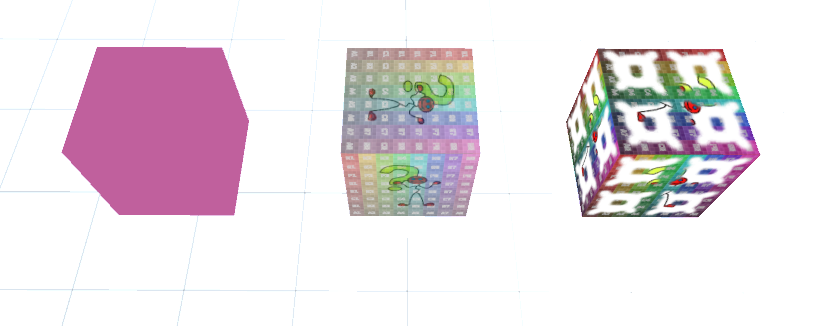## Interpolate Colors

The first version of this shader we’re exploring will just interpolate between two plain colors based on a value. Because of that we don’t need the variables connected to uv coordinates or textures for now, instead we add a second color variable and a simple value which will determine if the material shows the first of the second color. We define that blending property as a “Range” so we get a nice slider in the inspector.

``````//...

//show values to edit in inspector
Properties{
_Color ("Color", Color) = (0, 0, 0, 1) //the base color
_SecondaryColor ("Secondary Color", Color) = (1,1,1,1) //the color to blend to
_Blend ("Blend Value", Range(0,1)) = 0 //0 is the first color, 1 the second
}

//...

//the value that's used to blend between the colors
float _Blend;

//the colors to blend between
fixed4 _Color;
fixed4 _SecondaryColor;
``````

Apart from deleting the lines connected to UV coodinates, we can keep the vertex shader as it is. Instead we edit the fragment shader. As a first version we can just add the second color onto the first based on the blend value.

``````//the fragment shader
fixed4 frag(v2f i) : SV_TARGET{
fixed4 col = _Color + _SecondaryColor * _Blend;
return col;
}
``````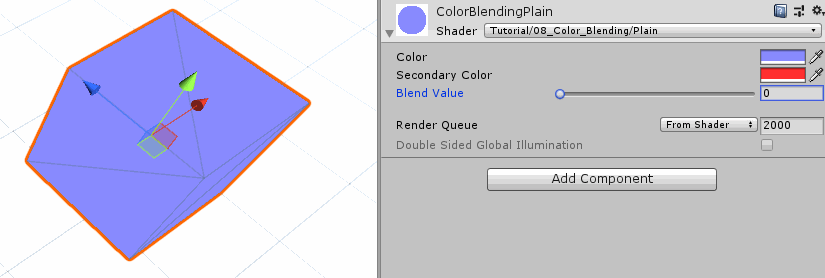We can already see that the color changes, but it doesn’t change to the secondary color. That’s because while the secondary color gets factored in, the primary color is still there (it’s similar to pointing two lights of different colors at one spot).

To fix this we can lessen the effect of the primary color as we increase the blend value. With a blend value of 0 we don’t see any of the secondary color and all of the primary one and with a blend value of 1 we want to see all of the secondary color and nothing of the primary color. To archive that, we multiply the primary color with one minus the blend value, turning 1 to 0 and 0 to 1.

``````//the fragment shader
fixed4 frag(v2f i) : SV_TARGET{
fixed4 col = _Color * (1 - _Blend) + _SecondaryColor * _Blend;
return col;
}
``````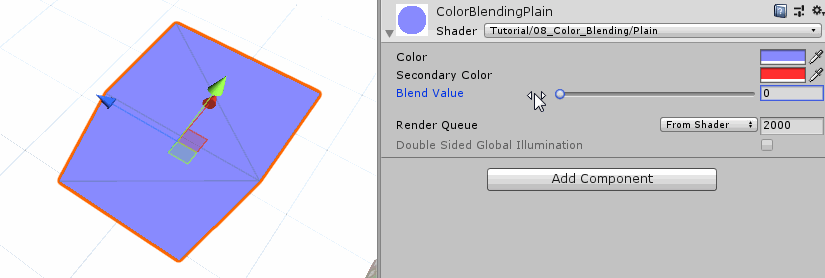This process is also called linear interpolation and theres a function built into hlsl that does this for us called lerp. It takes a value to interpolate from, a value to interpolate to and a interpolation value.

``````//the fragment shader
fixed4 frag(v2f i) : SV_TARGET{
fixed4 col = lerp(_Color, _SecondaryColor, _Blend);
return col;
}
``````

The complete shader for interpolating between two colors looks like this:

``````Shader "Tutorial/009_Color_Blending/Plain"{
//show values to edit in inspector
Properties{
_Color ("Color", Color) = (0, 0, 0, 1) //the base color
_SecondaryColor ("Secondary Color", Color) = (1,1,1,1) //the color to blend to
_Blend ("Blend Value", Range(0,1)) = 0 //0 is the first color, 1 the second
}

//the material is completely non-transparent and is rendered at the same time as the other opaque geometry
Tags{ "RenderType"="Opaque" "Queue"="Geometry"}

Pass{
CGPROGRAM

#include "UnityCG.cginc"

#pragma vertex vert
#pragma fragment frag

//the value that's used to blend between the colors
float _Blend;

//the colors to blend between
fixed4 _Color;
fixed4 _SecondaryColor;

//the object data that's put into the vertex shader
struct appdata{
float4 vertex : POSITION;
};

//the data that's used to generate fragments and can be read by the fragment shader
struct v2f{
float4 position : SV_POSITION;
};

v2f vert(appdata v){
v2f o;
//convert the vertex positions from object space to clip space so they can be rendered
o.position = UnityObjectToClipPos(v.vertex);
return o;
}

fixed4 frag(v2f i) : SV_TARGET{
fixed4 col = lerp(_Color, _SecondaryColor, _Blend);
return col;
}

ENDCG
}
}
}
``````

## Interpolate Textures

The next version of this shader will involve interpolating between colors we read from textures. For that we remove the color properties and variables to instead add properties and variables for two textures. We also introduce variables for uv coordinates again, but unlike in the texture tutorial we’re not applying the tiling and offset of the texture in the vertex shader. That’s because we have several textures that all use the same uv coodinates and we don’t want to interpolate all of them when we don’t have to.

``````//...

//show values to edit in inspector
Properties{
_MainTex ("Texture", 2D) = "white" {} //the base texture
_SecondaryTex ("Secondary Texture", 2D) = "black" {} //the texture to blend to
_Blend ("Blend Value", Range(0,1)) = 0 //0 is the first color, 1 the second
}

//...

//the object data that's put into the vertex shader
struct appdata{
float4 vertex : POSITION;
float2 uv : TEXCOORD0;
};

//the data that's used to generate fragments and can be read by the fragment shader
struct v2f{
float4 position : SV_POSITION;
float2 uv : TEXCOORD0;
};

v2f vert(appdata v){
v2f o;
//convert the vertex positions from object space to clip space so they can be rendered
o.position = UnityObjectToClipPos(v.vertex);
o.uv = v.uv;
return o;
}

//...
``````

Then, in the fragment shader, we can apply the tiling and offset separately for the two textures via the transform tex macro like we’re used to. Next we use those coordinates to read the two textures. After we did that we can use the colors we read from the textures and interpolate between them like we’re used to.

``````//the fragment shader
fixed4 frag(v2f i) : SV_TARGET{
//calculate UV coordinates including tiling and offset
float2 main_uv = TRANSFORM_TEX(i.uv, _MainTex);
float2 secondary_uv = TRANSFORM_TEX(i.uv, _SecondaryTex);

fixed4 main_color = tex2D(_MainTex, main_uv);
fixed4 secondary_color = tex2D(_SecondaryTex, secondary_uv);

//interpolate between the colors
fixed4 col = lerp(main_color, secondary_color, _Blend);
return col;
}
``````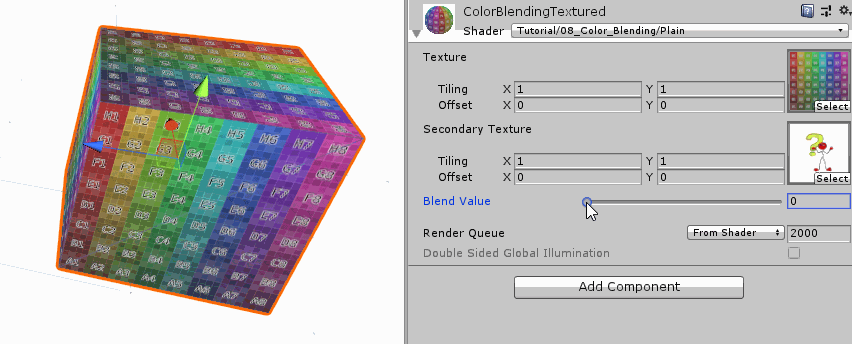The complete shader for interpolating between two textures looks like this:

``````Shader "Tutorial/009_Color_Blending/Texture"{
//show values to edit in inspector
Properties{
_MainTex ("Texture", 2D) = "white" {} //the base texture
_SecondaryTex ("Secondary Texture", 2D) = "black" {} //the texture to blend to
_Blend ("Blend Value", Range(0,1)) = 0 //0 is the first color, 1 the second
}

//the material is completely non-transparent and is rendered at the same time as the other opaque geometry
Tags{ "RenderType"="Opaque" "Queue"="Geometry"}

Pass{
CGPROGRAM

#include "UnityCG.cginc"

#pragma vertex vert
#pragma fragment frag

//the value that's used to blend between the colors
float _Blend;

//the colors to blend between
sampler2D _MainTex;
float4 _MainTex_ST;

sampler2D _SecondaryTex;
float4 _SecondaryTex_ST;

//the object data that's put into the vertex shader
struct appdata{
float4 vertex : POSITION;
float2 uv : TEXCOORD0;
};

//the data that's used to generate fragments and can be read by the fragment shader
struct v2f{
float4 position : SV_POSITION;
float2 uv : TEXCOORD0;
};

v2f vert(appdata v){
v2f o;
//convert the vertex positions from object space to clip space so they can be rendered
o.position = UnityObjectToClipPos(v.vertex);
o.uv = v.uv;
return o;
}

fixed4 frag(v2f i) : SV_TARGET{
//calculate UV coordinates including tiling and offset
float2 main_uv = TRANSFORM_TEX(i.uv, _MainTex);
float2 secondary_uv = TRANSFORM_TEX(i.uv, _SecondaryTex);

fixed4 main_color = tex2D(_MainTex, main_uv);
fixed4 secondary_color = tex2D(_SecondaryTex, secondary_uv);

//interpolate between the colors
fixed4 col = lerp(main_color, secondary_color, _Blend);
return col;
}

ENDCG
}
}
}
``````

## Interpolation based on a Texture

Lastly I’m going to show you a shader that doesn’t use one uniform variable to blend between the textures, but instead takes the blend value from a texture.

For this we start by deleting the variable and property we used for blending and instead add another texture.

``````//...

//show values to edit in inspector
Properties{
_MainTex ("Texture", 2D) = "white" {} //the base texture
_SecondaryTex ("Secondary Texture", 2D) = "black" {} //the texture to blend to
_BlendTex ("Blend Texture", 2D) = "grey" //black is the first color, white the second
}

//...

//the texture that's used to blend between the colors
sampler2D _BlendTex;
float4 _BlendTex_ST;

//the colors to blend between
sampler2D _MainTex;
float4 _MainTex_ST;

sampler2D _SecondaryTex;
float4 _SecondaryTex_ST;

//...
``````

We then also generate the transformed uv coordinates for that texture. With them, we read the color value from the texture. We now have a full color with red, green, blue and alpha components, but we want a simple 0-1 scalar value. To convert the color into a float we assume the texture is greyscale and just take out the red value of it. We then use this value to interpolate between the other two textures like we did before.

``````//the fragment shader
fixed4 frag(v2f i) : SV_TARGET{
//calculate UV coordinates including tiling and offset
float2 main_uv = TRANSFORM_TEX(i.uv, _MainTex);
float2 secondary_uv = TRANSFORM_TEX(i.uv, _SecondaryTex);
float2 blend_uv = TRANSFORM_TEX(i.uv, _BlendTex);

fixed4 main_color = tex2D(_MainTex, main_uv);
fixed4 secondary_color = tex2D(_SecondaryTex, secondary_uv);
fixed4 blend_color = tex2D(_BlendTex, blend_uv);

//take the red value of the color from the blend texture
fixed blend_value = blend_color.r;

//interpolate between the colors
fixed4 col = lerp(main_color, secondary_color, blend_value);
return col;
}
``````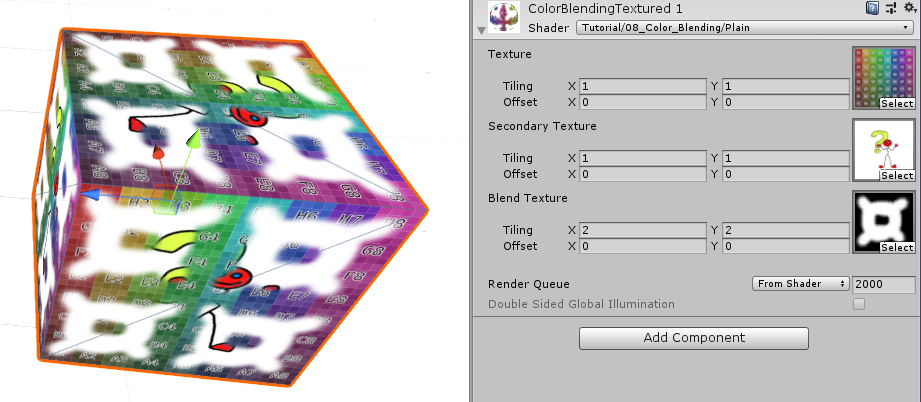The complete shader for interpolating based on a texture looks like this:

``````Shader "Tutorial/009_Color_Blending/TextureBasedBlending"{
//show values to edit in inspector
Properties{
_MainTex ("Texture", 2D) = "white" {} //the base texture
_SecondaryTex ("Secondary Texture", 2D) = "black" {} //the texture to blend to
_BlendTex ("Blend Texture", 2D) = "grey" //black is the first color, white the second
}

//the material is completely non-transparent and is rendered at the same time as the other opaque geometry
Tags{ "RenderType"="Opaque" "Queue"="Geometry"}

Pass{
CGPROGRAM

#include "UnityCG.cginc"

#pragma vertex vert
#pragma fragment frag

//the texture that's used to blend between the colors
sampler2D _BlendTex;
float4 _BlendTex_ST;

//the colors to blend between
sampler2D _MainTex;
float4 _MainTex_ST;

sampler2D _SecondaryTex;
float4 _SecondaryTex_ST;

//the object data that's put into the vertex shader
struct appdata{
float4 vertex : POSITION;
float2 uv : TEXCOORD0;
};

//the data that's used to generate fragments and can be read by the fragment shader
struct v2f{
float4 position : SV_POSITION;
float2 uv : TEXCOORD0;
};

v2f vert(appdata v){
v2f o;
//convert the vertex positions from object space to clip space so they can be rendered
o.position = UnityObjectToClipPos(v.vertex);
o.uv = v.uv;
return o;
}

fixed4 frag(v2f i) : SV_TARGET{
//calculate UV coordinates including tiling and offset
float2 main_uv = TRANSFORM_TEX(i.uv, _MainTex);
float2 secondary_uv = TRANSFORM_TEX(i.uv, _SecondaryTex);
float2 blend_uv = TRANSFORM_TEX(i.uv, _BlendTex);

fixed4 main_color = tex2D(_MainTex, main_uv);
fixed4 secondary_color = tex2D(_SecondaryTex, secondary_uv);
fixed4 blend_color = tex2D(_BlendTex, blend_uv);

//take the red value of the color from the blend texture
fixed blend_value = blend_color.r;

//interpolate between the colors
fixed4 col = lerp(main_color, secondary_color, blend_value);
return col;
}

ENDCG
}
}
}
``````

I hope this tutorial helped you understand how to work with colors in shaders and interpolation in particular.

You can find the source code to the shaders here:

You can also find me on twitter at @totallyRonja. If you liked my tutorial and want to support me you can do that on Patreon (patreon.com/RonjaTutorials) or Ko-Fi (ko-fi.com/RonjaTutorials).